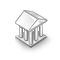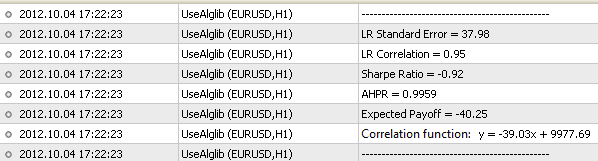Interesting script?
So post a link to it -
let others appraise it
You liked the script? Try it in the MetaTrader 5 terminal# ALGLIB - Numerical Analysis Library - library for MetaTrader 5

Sergey Bochkanov |
Views:
38104
Rating:
Published:
2012.10.12 08:48
Updated:
2019.09.03 16:26

Real author:

Sergey Bochkanov. ALGLIB project website - http://www.alglib.net/. The library dates back to 1999.

### ALGLIB is one of the largest and most complete mathematical libraries

Do you need to make a fast Fourier transform or to solve a differential equation system? Do you perform a complex data analysis trying to gather all methods in one place as a source code? Then ALGLIB library of numerical methods is for you!

ALGLIB is currently one of the best libraries of the multi-language algorithms. Below are ALGLIB features mentioned on the official website:

ALGLIB is a cross-platform numerical analysis and data processing library. It supports several programming languages (C++, C#, Pascal, VBA) and several operating systems (Windows, Linux, Solaris). ALGLIB features include:

• Linear algebra (direct algorithms, EVD/SVD)
• Solvers (linear and nonlinear)
• Interpolation
• Optimization
• Fast Fourier transforms
• Numerical integration
• Linear and nonlinear least-squares fitting
• Ordinary differential equations
• Special functions
• Statistics (descriptive statistics, hypothesis testing)
• Data analysis (classification/regression, including neural networks)
• Multiple precision versions of linear algebra, interpolation optimization and others algorithms (using MPFR for floating point computations)

Why to choose ALGLIB? Because it is:

• portable. It can be compiled almost anywhere using almost any compiler (see compatibility matrix for more info).
• easy to use. It supports many programming languages. If you use one language, you don't need to study another (FORTRAN, for example) to compile and link an external library.
• open source. It can be used for free under GPL 2+.
• suited for commercial users too. Those who want to use ALGLIB in commercial applications can buy commercial license without copyleft requirement.

ALGLIB library is being constantly enhanced, new functions and improvements according to users' comments are implemented regularly. The latest version is 3.6.0.

Besides, the library contains the large collection of test cases covering the major part of the proposed methods' functionality. That will allow you to carry the tests and report detected errors to the project's authors.

CAlglib class static functions should be used to work with the library - all library functions are moved to CAlglib system class as static functions.

testclasses.mq5 and testinterfaces.mq5 test cases scripts are attached together with a simple usealglib.mq5 demo script. Include files of the same name (testclasses.mqh and testinterfaces.mqh) are used to launch test cases. They should be placed to \MQL5\Scripts\Alglib\Testcases\.

Note: testclasses.mq5 script execution takes quite a long time (about 30 minutes).

Below is more detailed information about ALGLIB MQL5 ported library packages:

Packages
Description
alglib.mqh
The main library package includes custom functions. These functions should be called for working with the library.
alglibinternal.mqh
Additional classes for the operation of other library packages.
alglibmisc.mqh

The package contains the following classes:

1. CHighQualityRand - high quality random number generator.
2. CNearestNeighbor - KD-trees generation and solution.
ap.mqh The package also contains additional classes necessary for other packages.
bitconvert.mqh С++ basic classes and functions that are absent in MQL5 as built-in:
1. BitConverter - class for transforming int and double type figures into an array of bits and vice versa.
2. CInfOrNaN - NaNs and plus/minus infinity verification and generation class.
3. ArrayReverse - array elements reverse function.
4. GetSelectionString - function for receiving the string generated from char type array elements.
5. MathSign - number sign calculation function.
6. MathSinh - hyperbolic sine calculation function.
7. MathCosh - hyperbolic sine calculation function.
8. MathTanh - hyperbolic tangent calculation function.
complex.mqh Defining complex structures for working with complex numbers.
dataanalysis.mqh Classes of data analysis:
1. CBdSS - error functions calculation.
2. CDForest - working with the forests of solution trees.
3. CKMeans - clustering using k-means++ algorithm.
4. CLDA - linear discriminant analysis.
5. CLinReg - linear regression.
6. CMLPBase - multilayer perceptron (neural networks).
7. CLogit - multinomial logit regression.
8. CMarkovCPD - Markov chains for population data.
9. CMLPTrain - training of multilayer perceptron.
10. CMLPE - ensembles of neural networks.
11. CPCAnalysis - principal components method.
delegatefunctions.mqh The package contains the classes created as replacement for the delegates. The objects of these classes are the functions optimized in several library methods.
diffequations.mqh The class for solving ordinary differential equations:
1. CODESolver - solving ordinary differential equations.
fasttransforms.mqh Fast transformation classes:
1. CFastFourierTransform - fast Fourier transform.
2. CConv - convolution.
3. CCorr - cross-correlation.
4. CFastHartleyTransform - fast Hartley transform.
integration.mqh Classes for numerical integration:
2. CGaussKronrodQ - Gauss–Kronrod quadrature formulas.
interpolation.mqh Interpolation, approximation and numerical differentiation classes:
1. CIDWInt - interpolation and approximation by the inverse average weighted distance.
2. CRatInt - rational interpolation.
3. CPolInt - polynomial interpolation.
4. CSpline1D - one-dimensional spline interpolation.
5. CLSFit - approximation using linear or non-linear least squares method.
6. CPSpline - parametric spline interpolation.
7. CSpline2D - two-dimensional spline interpolation.
linalg.mqh Classes for calculation of some linear algebra operations:
1. COrtFac - QR/LQ decompositions, decompositions of Hessenberg, as well as of bi- and tridiagonal matrices.
2. CEigenVDetect - finding the eigenvalues ​​and eigenvectors.
3. CMatGen - random matrices generation.
4. CTrFac - LU and Cholesky decompositions.
5. CRCond - estimation of a matrix dependence value.
6. CMatInv - matrix inversion.
7. CBdSingValueDecompose - singular decomposition of a bidiagonal matrix.
8. CSingValueDecompose - matrix singular decomposition.
9. CFbls - quick basic linear solutions.
10. CMatDet - calculation of a matrix determinant.
11. CSpdGEVD - finding the eigenvalues ​​and eigenvectors in generalized symmetric matrices.
12. CInverseUpdate - matrices inversion and update.
13. CSchur - Issai Schur decomposition.
matrix.mqh Classes of matrices: integer, continuous and complex.
optimization.mqh Dimensional and multidimensional optimization classes:
1. CMinCG - optimization using conjugate gradient method.
2. CMinBLEIC - optimization with linear constraints in the form of equations and inequations.
3. CMinLBFGS - optimization using successive generation and classification of the function's quadratic model.
4. CMinQP - quadratic programming with linear constraints in the form of equations and inequations.
5. CMinLM - optimization using Levenberg-Marquardt algorithm.
6. CMinComp - backward compatibility functions.
solvers.mqh Classes for solving linear and non-linear equation systems:
1. CDenseSolver - solving linear equation systems.
2. CNlEq - solving non-linear equation systems.
specialfunctions.mqh Classes of distribution functions, integrals and polynomials:
1. CGammaFunc - Gamma function.
2. CIncGammaF - incomplete Gamma function.
3. CBetaF - Beta function.
4. CIncBetaF - incomplete beta function.
5. CPsiF - psi-function.
6. CAiryF - Airy function.
7. CBessel - Bessel functions of integral order.
8. CJacobianElliptic - Jacobian elliptic functions.
9. CDawson - Dawson integral.
10. CTrigIntegrals - trigonometric integrals.
11. CElliptic - elliptic integrals of the first and second kind.
12. CExpIntegrals - exponential integrals.
13. CFresnel - Fresnel integrals.
14. CHermite - Hermite polynomials.
15. CChebyshev - Chebyshev polynomials.
16. CLaguerre - Laguerre polynomials.
17. CLegendre - Legendre polynomials.
18. CChiSquareDistr - chi-square distribution.
19. CBinomialDistr - binomial distribution.
20. CNormalDistr - normal distribution.
21. CPoissonDistr - Poisson distribution.
22. CStudenttDistr - Student t-distribution.
23. CFDistr - F-distribution.
statistics.mqh Classes of statistical data analysis:
1. CBaseStat - basic statistical methods.
2. CCorrTests - test for correlation ratio significance.
3. CJarqueBera - JarqueBera croterion.
4. CMannWhitneyU - Mann-Whitney U-criterion.
5. CSignTest - sign test.
6. CStudentTests - Student t-tests.
7. CVarianceTests - F-test and chi-square test.
8. CWilcoxonSignedRank - Wilcoxon W-criterion.

Code:

Library functions have detailed comments on their usage.

```//+------------------------------------------------------------------+
//| Calculation of the distribution moments: mean, variance,         |
//| skewness, kurtosis.                                              |
//| INPUT PARAMETERS:                                                |
//|     X       -   sample                                           |
//|     N       -   N>=0, sample size:                               |
//|                 * if given, only leading N elements of X are     |
//|                   processed                                      |
//|                 * if not given, automatically determined from    |
//|                   size of X                                      |
//| OUTPUT PARAMETERS                                                |
//|     Mean    -   mean.                                            |
//|     Variance-   variance.                                        |
//|     Skewness-   skewness (if variance<>0; zero otherwise).       |
//|     Kurtosis-   kurtosis (if variance<>0; zero otherwise).       |
//+------------------------------------------------------------------+
static bool CBaseStat::SampleMoments(const double &cx[],const int n,double &mean,
double &variance,double &skewness,double &kurtosis)
{
//--- check
if(!CAp::Assert(n>=0,__FUNCTION__+": the error variable"))
return(false);
//--- check
if(!CAp::Assert(CAp::Len(cx)>=n,__FUNCTION__+": length(x)<n"))
return(false);
//--- check
if(!CAp::Assert(CApServ::IsFiniteVector(cx,n),__FUNCTION__+": x is not finite vector"))
return(false);
//--- create variables
double v=0;
double v1=0;
double v2=0;
double stddev=0;
//--- Init, special case 'N=0'
mean=0;
variance=0;
skewness=0;
kurtosis=0;
//--- check
if(n<=0)
return(true);
//--- Mean
for(int i=0;i<n;i++)
mean+=cx[i];
mean/=n;
//--- Variance (using corrected two-pass algorithm)
if(n!=1)
{
//--- calculation
for(int i=0;i<n;i++)
v1+=CMath::Sqr(cx[i]-mean);
for(int i=0;i<n;i++)
v2+=cx[i]-mean;
v2=CMath::Sqr(v2)/n;
variance=(v1-v2)/(n-1);
//--- calculation
stddev=MathSqrt(variance);
}
else
variance=EMPTY_VALUE;
//--- Skewness and kurtosis
if(stddev!=0)
{
//--- calculation
for(int i=0;i<n;i++)
{
v=(cx[i]-mean)/stddev;
v2=CMath::Sqr(v);
skewness+=v2*v;
kurtosis+=CMath::Sqr(v2);
}
//--- change values
skewness=skewness/n;
kurtosis=kurtosis/n-3;
}
//--- successful execution
return(true);
}```

CAlglib class static functions should be used to work with the library. Below is usealglib.mq5 function script's source code for calculation of some statistical tading parameters:

```//+------------------------------------------------------------------+
//|                                                    UseAlglib.mq5 |
//|                        Copyright 2012, MetaQuotes Software Corp. |
//|                                              https://www.mql5.com |
//+------------------------------------------------------------------+
#property version   "1.00"
//+------------------------------------------------------------------+
//| Connecting the libraries                                         |
//+------------------------------------------------------------------+
#include <Math\Alglib\alglib.mqh>
#include <Arrays\ArrayDouble.mqh>
//+------------------------------------------------------------------+
//| Script program start function                                    |
//+------------------------------------------------------------------+
void OnStart()
{
//--- object to access data on deals
CDealInfo     deal;
//--- object for storing profit/loss of each deal
CArrayDouble *profit=new CArrayDouble;
//--- objects for storing the balance
CArrayDouble *balance_total=new CArrayDouble;
//--- initial balance
double        balance=0;
HistorySelect(0,TimeCurrent());
//--- total amount of deals
int deals_total=HistoryDealsTotal();
//--- receive data on deals' profit and balance
for(int i=0;i<deals_total;i++)
{
//--- move to the deal having i index
deal.SelectByIndex(i);
if(deal.DealType()==DEAL_TYPE_BALANCE)
{
if(NormalizeDouble(deal.Profit()+deal.Swap(),2)>=0.0)
if(balance==0.0)
balance=deal.Profit();
}
if(deal.Entry()==DEAL_ENTRY_OUT || deal.Entry()==DEAL_ENTRY_INOUT)
{
balance=balance+NormalizeDouble(deal.Profit()+deal.Swap()+deal.Commission(),2);
}
}
//--- copy data on the balance to double type array
double arr_balance[];
ArrayResize(arr_balance,balance_total.Total());
for(int i=0;i<balance_total.Total();i++)
arr_balance[i]=balance_total.At(i);
//--- copy data on profit to double type array
double arr_profit[];
ArrayResize(arr_profit,profit.Total());
for(int i=0;i<profit.Total();i++)
arr_profit[i]=profit.At(i);
//--- linear regression
//--- number of independent variables
int nvars=1;
//--- sample volume
int npoints=balance_total.Total();
//--- create the matrix of parameters for the linear regression
CMatrixDouble xy(npoints,nvars+1);
for(int i=0;i<npoints;i++)
{
xy[i].Set(0,i);
xy[i].Set(1,arr_balance[i]);
}
//--- variable for detecting calculations result (successful, unsuccessful)
int info;
//--- class objects necessary for storing data on calculations
CLinearModelShell lm;
CLRReportShell    ar;
//--- arrays for storing regression results
double lr_coeff[];
double lr_values[];
ArrayResize(lr_values,npoints);
//--- calculate linear regression ratios
CAlglib::LRBuild(xy,npoints,nvars,info,lm,ar);
CAlglib::LRUnpack(lm,lr_coeff,nvars);
//--- receive recovered linear regression values
for(int i=0;i<npoints;i++)
lr_values[i]=lr_coeff*i+lr_coeff;
//--- calculate Expected Payoff
double exp_payoff,tmp1,tmp2,tmp3;
CAlglib::SampleMoments(arr_profit,exp_payoff,tmp1,tmp2,tmp3);
//--- calculate HPR array
double HPR[];
ArrayResize(HPR,balance_total.Total()-1);
for(int i=0;i<balance_total.Total()-1;i++)
HPR[i]=balance_total.At(i+1)/balance_total.At(i);
//--- calculate standard deviation and math expectation from HPR
double AHPR,SD;
CAlglib::SampleMoments(HPR,AHPR,SD,tmp2,tmp3);
SD=MathSqrt(SD);
//--- calculate LR Correlation
double lr_corr=CAlglib::PearsonCorr2(arr_balance,lr_values);
double lr_stand_err=0;
for(int i=0;i<npoints;i++)
{
double delta=MathAbs(arr_balance[i]-lr_values[i]);
lr_stand_err=lr_stand_err+delta*delta;
}
lr_stand_err=MathSqrt(lr_stand_err/(npoints-2));
//--- calculate Sharpe Ratio
double sharpe_ratio=(AHPR-1)/SD;
//--- print
PrintFormat("-----------------------------------------------");
PrintFormat("Correlation function: y = %.2fx + %.2f",lr_coeff,lr_coeff);
//--- parameters
PrintFormat("Expected Payoff = %.2f",exp_payoff);
PrintFormat("AHPR = %.4f",AHPR);
PrintFormat("Sharpe Ratio = %.2f",sharpe_ratio);
PrintFormat("LR Correlation = %.2f",lr_corr);
PrintFormat("LR Standard Error = %.2f",lr_stand_err);
PrintFormat("-----------------------------------------------");
//--- delete objects
delete profit;
delete balance_total;
}
//+------------------------------------------------------------------+
```Translated from Russian by MetaQuotes Ltd.
Original code: https://www.mql5.com/ru/code/1146Ask Bid Ticks

Ask Bid Ticks is a high-precision, real-time tick data solution for microstructure analysis (a tick data collector). It works with local computer time.Daily Range

This indicator calculates and shows daily highest and lowest prices, display them as two set of lines.AML Adaptive Market Level

Adaptive Market Level shows the current reference level of the market price. The level is shifted only in case of the price trend movement.BubblesAndDrops

The indicator shows possible price movement direction.